Home | Search | About | Fidelio | Economy | Strategy | Justice | Conferences | Join Highlights | Calendar | Music | Books | Concerts | Links | Education | Health What's New | LaRouche | Spanish Pages | Poetry | Maps Dialogue of Cultures SCHILLER INSTITUTE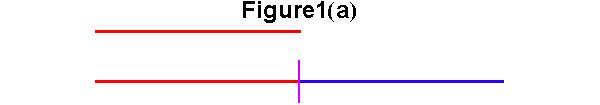1(a) The magnitude which has the "power" to double the length of a line is produced by a simple extension of a line.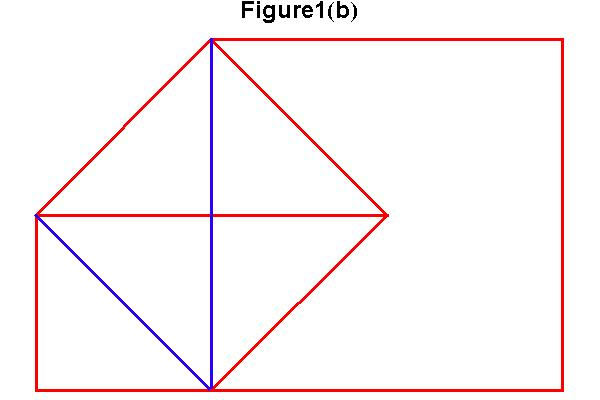1(b) The magnitude which has the "power" to produce a square of double area, is the diagonal of the smaller square, and is called "the geometric mean," between the two squares. The magnitude of the length of the diagonal is incommensurable to the magnitude of the length of the line.1(c) The magnitude which has the "power" to produce a cube whose volume is double, is different than the magnitude which has the "power" to double a square or a line. That magnitude is the smaller of two geometric means between the two cubes. This magnitude is incommensurable to both those lower powers.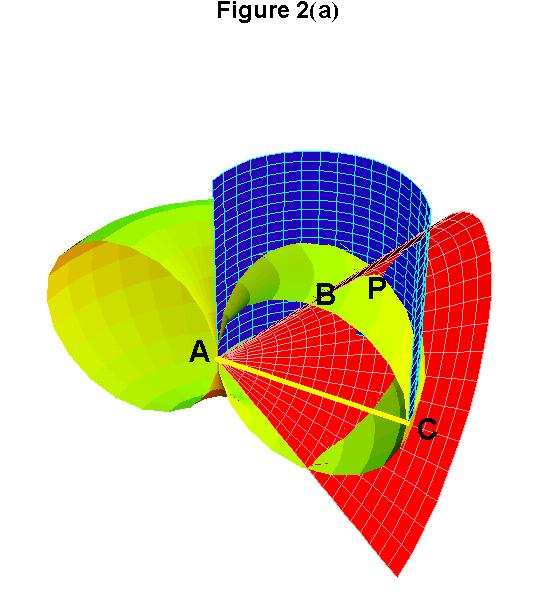2(a) Archytus developed a construction to find two geometric means between two magnitudes. The longer magnitude is AC, which is the diameter of a circle. That circle is rotated around A to forms a torus. A cylinder is then produced perpendicular to the torus, whose diameter is also AC. The shorter magnitude AB is drawn as a chord of a cross section of the torus. AB is extended until it intersects the cylinder, forming a triangle, which when rotated produces a cone. All three surfaces intersect at point P.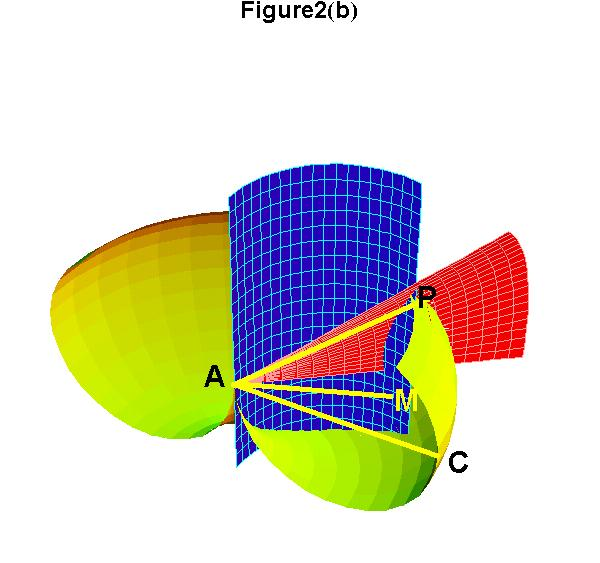2(b) This is a "cut-a-way" view of 2(a). M is found by dropping a line from P until it intersects the plane of the cylinder that includes AC. Archytus demonstrated that AM is the smaller mean between AB and AC, and AP is the longer mean. Thus, AB : AM :: AM:AP :: AP:AC. So, if AB = 1 and AC = 2, then AM = 21/3 and AP = 22/3 .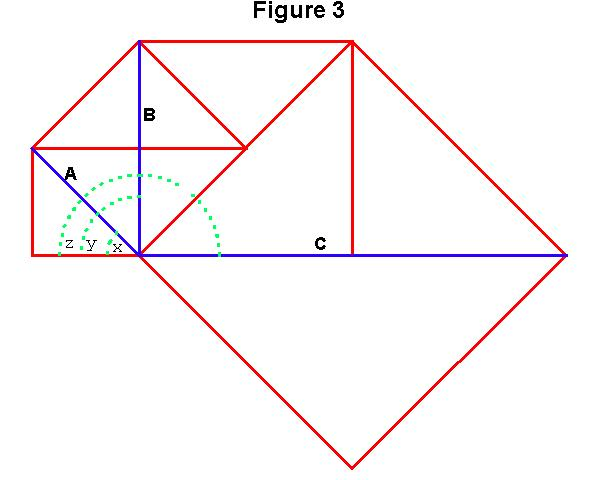3. The principle of 'squaring' involves doubling the angle of rotation and squaring the length. Angle y is double angle x. Angle z is double angle y. Also, the length of B is the square of A and the length of C is the square of B.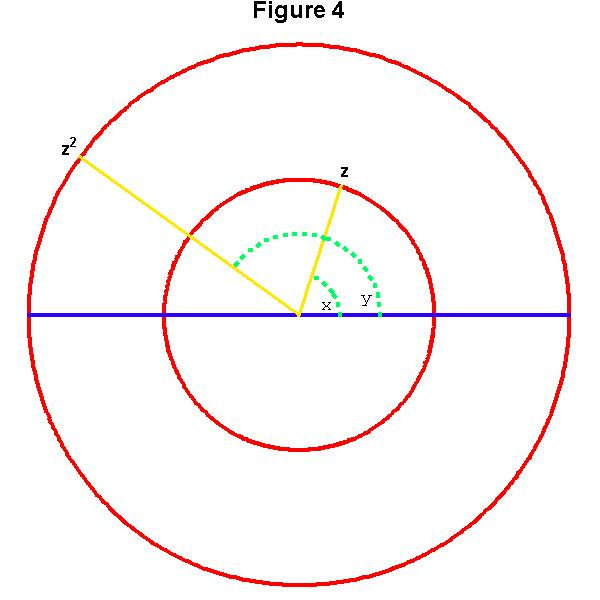4. The general principle of "squaring" can be carried out on a circle. z2 is produced from z by doubling angle x to angle y and squaring the distance from z to the center of the circle.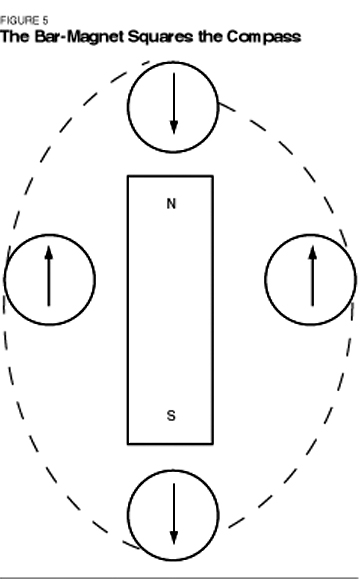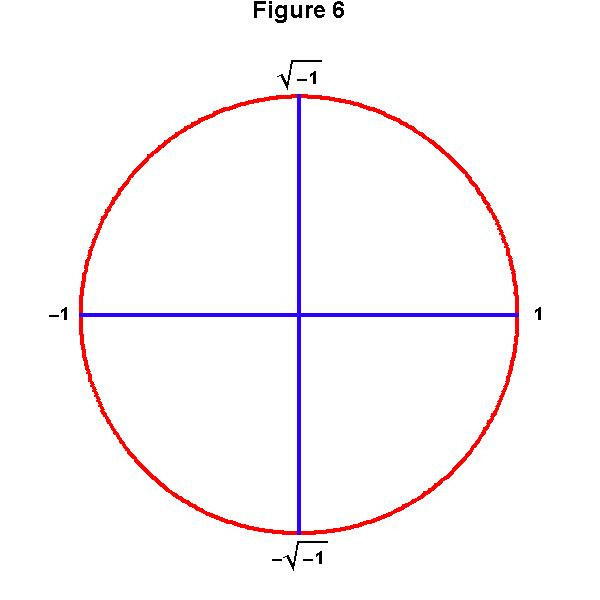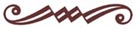schiller@schillerinstitute.org The Schiller Institute PO BOX 20244 Washington, DC 20041-0244 703-297-8368 Thank you for supporting the Schiller Institute. Your membership and contributions enable us to publish FIDELIO Magazine, and to sponsor concerts, conferences, and other activities which represent critical interventions into the policy making and cultural life of the nation and the world. Contributions and memberships are not tax-deductible. VISIT THESE OTHER PAGES: